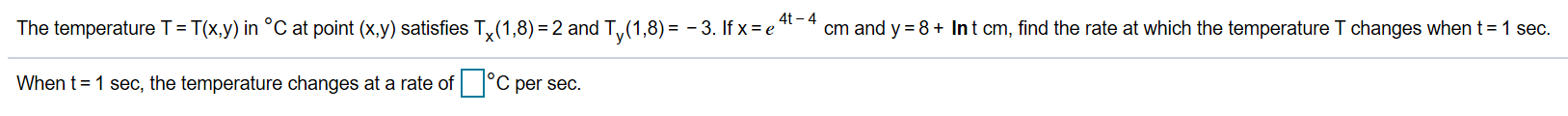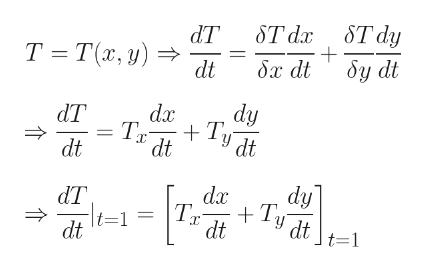# 4t-4cm and y 8+ Int cm, find the rate at which the temperature T changes when tThe temperature T= T(x.y) in °C at point (x.y) satisfies T,x(1,8) 2 and Ty(1,8)= -3. If x e'1 sec.C per sec.When t 1 sec, the temperature changes at a rate of

Question

I think this question (included in the image) is looking for a derivative of sortshelp_outlineImage Transcriptionclose4t-4 cm and y 8+ Int cm, find the rate at which the temperature T changes when t The temperature T= T(x.y) in °C at point (x.y) satisfies T,x(1,8) 2 and Ty(1,8)= -3. If x e' 1 sec. C per sec. When t 1 sec, the temperature changes at a rate of fullscreen
check_circleExpert Solution
Step 1

Please see the white board for the formulation.help_outlineImage TranscriptioncloseST dy ST dx Т -Та, у) бу dt бх dt dt dy dx dT + Tу dt dt dt dy da dT + Tу dt dt t=1 = dt t=1 fullscreen
Step 2

Step 3

y = 8+ ln t

Hence, dy/dt = 1/t

when t = 1, y = ...

### Want to see the full answer?

See Solution

#### Want to see this answer and more?

Solutions are written by subject experts who are available 24/7. Questions are typically answered within 1 hour*

See Solution
*Response times may vary by subject and question
Tagged in

### Calculus